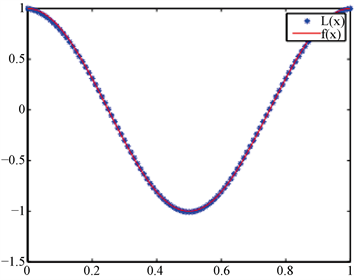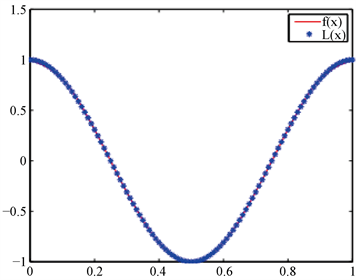﻿ 基于径向基函数插值的非线性积分方程求解

# 基于径向基函数插值的非线性积分方程求解Nonlinear Integral Equation Solution Based on Radial Basis Function Interpolation

Abstract: The radial basis function (RBF) interpolation is applied to the solution of the nonlinear integral equation. In this paper, the multiquadric (MQ) function with excellent interpolation performance in RBF is selected. First, the function to be solved is expressed as a linear combination of RBF, and then the equation is discretized into a non-linear square by collocation method. The approximate expression of the function to be solved is given after the weight coefficients obtained. Solving nonlinear integral equation based on MQ function interpolation can obtain higher accuracy with fewer nodes, which can be extended to high-dimensional integral equation. In this paper, we prove the existence and uniqueness of MQ basis function interpolation problem, and analyse the interpolation basis function of constructing non-linear function of MQ function by numerical examples, and obtain the ideal approximation effect and result.

1. 引言

$f\left(P\right)=\mu {\int }_{\Omega }k\left(P,Q\right)F\left[f\left(Q\right)\right]\text{d}Q+g\left(P\right),\text{\hspace{0.17em}}\text{\hspace{0.17em}}P,Q\in \Omega$ (1)

2. 基于RBF插值改进的配置法算法

2.1. RBF插值原理

$\Phi \left(x\right)={\int }_{{\Re }^{l}}{\text{e}}^{ixt}\stackrel{^}{\Phi }\left(t\right)\text{d}t$，当 ${x}_{j}$ 两两不同且 ${\lambda }_{j}$ 不全为零时，有

$\underset{j,k=1}{\overset{n}{\sum }}{\lambda }_{j}{\lambda }_{k}\Phi \left(‖x‖\right)={\int }_{{\Re }^{l}}|\underset{j}{\overset{n}{\sum }}{\lambda }_{j}{\text{e}}^{ix{j}^{t}}|\stackrel{^}{\Phi }\left(t\right)\text{d}t$ (2)

(1) $\psi \left(r\right)\in C\left[0,\infty \right]$

(2) $\psi \left(r\right)\in {C}^{\infty }\left[0,\infty \right]$

(3) ${\left(-1\right)}^{k}{\psi }^{k}\left(r\right)>0,\text{\hspace{0.17em}}r>0,\text{\hspace{0.17em}}k=0,1,2,\cdots$

$\underset{j,k=1}{\overset{n}{\sum }}{\lambda }_{j}{\lambda }_{k}\Phi \left(‖{x}_{k}-{x}_{j}‖\right)={\int }_{{\Re }^{l}}|\underset{j=1}{\overset{n}{\sum }}{\lambda }_{j}{\text{e}}^{ix{j}^{t}}|\stackrel{^}{\Phi }\left(t\right)\text{d}t>0$ (3)

$A=\left[\begin{array}{cc}\Phi \left(‖{x}_{j}-{x}_{k}‖\right)& \left({x}_{j}^{\beta }\right)\\ {\left({x}_{j}^{\beta }\right)}^{\text{T}}& 0\end{array}\right]$

${f}^{*}\left(x\right)=\underset{j=1}{\overset{n}{\sum }}{a}_{j}\Phi \left(‖x-{x}_{j}‖\right)+{\underset{|\beta | (4)

$f\left(x\right)={f}^{*}\left({x}_{k}\right),k=1,2,\cdots ,n$ (5)

2.2. 基于RBF插值的改进配置法算法

RBF是仅依赖于距离 $r=‖x-{x}_{j}‖$ 的函数，在多种RBF的定义中，常用的有薄板样条函数 $\Phi \left(r\right)={r}^{2}\mathrm{ln}r$ 高斯函数 $\Phi \left(r\right)={\text{e}}^{\beta {r}^{2}}$ 、Hardy提岀的多重二次曲面(multi-quadric, MQ)函数及多种紧支撑函数等。Franke在1982年证明了MQ方法是所有插值方法中综合性能最优的，因此本文选用MQ作为插值基函数，其常用表达式为

$\Phi \left(x\right)=\sqrt{{‖x-c‖}^{2}+{a}^{2}}$ (6)

Step 1：设置RBF函数的中心点c，形状参数 $\alpha \left(\alpha \in \left(0,1\right]\right)$，配置点 ${x}_{m},\text{\hspace{0.17em}}m=1,2,\cdots ,N$

Step 2：对给定的一组数据点 $\left({x}_{m},f\left({x}_{m}\right)\right)$，由RBF构造插值函数

$f\left(x\right)=\underset{i=1}{\overset{N}{\sum }}{\lambda }_{i}{\Phi }_{i}\left(x\right)=\underset{i=1}{\overset{N}{\sum }}{\lambda }_{i}\sqrt{{\left(x-{c}_{i}\right)}^{2}+{\alpha }^{\text{2}}}$ (7)

Step 3：将插值点代入(7)式，得

$f\left({x}_{m}\right)=\underset{i=1}{\overset{N}{\sum }}{\lambda }_{i}{\Phi }_{i}\left({x}_{m}\right)=\underset{i=1}{\overset{N}{\sum }}{\lambda }_{i}\sqrt{{\left({x}_{m}-{c}_{i}\right)}^{2}+{\alpha }^{2}}$ (8)

$\left[{\Phi }_{d}\right]\left[\lambda \right]=\left[{f}_{d}\right]$ (9)

Step 4: 矩阵元素计算式为 ${\Phi }_{mn}=\sqrt{{\left({x}_{m}-{c}_{n}\right)}^{2}+{\alpha }^{2}}$，求解(9)式得

$\left[\lambda \right]={\left[{\Phi }_{d}\right]}^{-1}\left[{f}_{d}\right]$ (10)

Step 5: 得岀函数 $f\left(x\right)$ 的RBF逼近形式

$f\left(x\right)=\left[\Phi \left(x\right)\right]\left[\lambda \right]=\left[\Phi \left(x\right)\right]{\left[{\Phi }_{d}\right]}^{-1}\left[{f}_{d}\right]$ (11)

3. Multi-Quadric函数插值的存在性与唯一性

$S\left(x\right)={\sum }_{j=1}^{n}\Phi \left(‖{x}_{k}-{x}_{j}‖\right)$

$S\left({x}_{k}\right)=\underset{j=1}{\overset{n}{\sum }}{\lambda }_{j}\Phi \left(‖{x}_{k}-{x}_{j}‖\right)={f}_{k},k=1,2,\cdots ,n$

$\sum \sum {\lambda }_{j}{\lambda }_{k}{\text{e}}^{-{‖{x}_{j}-{x}_{k}‖}^{2}}={\left(2\pi \right)}^{d/2}\int {|{\lambda }_{j}{\text{e}}^{ix{j}^{\theta }}|}^{2}{\text{e}}^{-{‖\theta ‖}^{1/2}}\text{d}\theta >0$

$\begin{array}{l}\sum {\lambda }_{j}{\lambda }_{k}{\int }_{0}^{\infty }{\theta }^{-3/2}\left(1-{\text{e}}^{-\theta \left({\alpha }^{2}+{‖x‖}^{2}\right)}\right)\text{d}\theta \\ =\sum {\lambda }_{j}{\lambda }_{k}{\left({\alpha }^{2}+{‖{x}_{k}-{x}_{j}‖}^{2}\right)}^{1/2}{\int }_{0}^{\infty }{\theta }^{-3/2}\left(1-{\text{e}}^{-\theta }\right)\text{d}\theta \\ =h\sum {\lambda }_{j}{\lambda }_{k}{\left({\alpha }^{2}+{‖{x}_{k}-{x}_{j}‖}^{2}\right)}^{1/2}\\ <0\end{array}$

$\sum {\lambda }_{j}{\lambda }_{k}{\left({\alpha }^{2}+{‖{x}_{k}-{x}_{j}‖}^{2}\right)}^{1/2}<0$

$\sum {\lambda }_{j}{\lambda }_{k}{\left({\alpha }^{2}+{‖{x}_{k}-{x}_{j}‖}^{2}\right)}^{1/2}={\sum \left({\alpha }^{2}+{‖{x}_{k}-{x}_{j}‖}^{2}\right)}^{1/2}>0$

4. 数值实验及分析

$L\left(x\right)=\underset{j=0}{\overset{N}{\sum }}{a}_{j}{\Phi }_{j}\left(x\right)$(12)

$L\left({x}_{k}\right)=\underset{j=0}{\overset{N}{\sum }}{a}_{j}{\Phi }_{j}\left({x}_{k}\right)=\underset{j=0}{\overset{N}{\sum }}{a}_{j}\left({x}_{j}-{x}_{k}\right)=f\left({x}_{k}\right)$ (13)

$\Phi \left({x}_{j}-{x}_{k}\right)=\sqrt{{\alpha }^{2}+{\left({x}_{j}-{x}_{k}\right)}^{2}}\left(k=0,1,\cdots ,N;j=0,1,\cdots ,N\right)$ 非奇异，故此方程组有唯一解，可求出 ${a}_{j}$，代入(12)式得到 $L\left(x\right)$，进而求出插值函数。下面给出了误差表1及两种插值图1图2Figure 1. $N=6$, $L\left(x\right),f\left(x\right)$ function imageFigure 2. $N=11$, $L\left(x\right),f\left(x\right)$ function imageTable 1. The maximum error comparison between the method in this paper and the methods in   

5. 结论

NOTES

*通讯作者。

 Rabbani, M., Maleknejad, K., Aghazadeh, N. and Mollapourasl, R. (2007) Computational Projection Methods for Solving Fredholm Integral Equation. Applied Mathematics and Computation, 191, 140-143.
https://doi.org/10.1016/j.amc.2007.02.071

 Aziz, I. and Sirai-ul-islam (2013) New Algorithms for the Numerical Solution of Nonlinear Fredholm and Volterra Integral Equations Using Haar Wavelets. Journal of Computational and Applied Mathematics, 239, 333-345.
https://doi.org/10.1016/j.cam.2012.08.031

 Babolian, E., Bazm, S. and Lima, P. (2011) Numerical Solution of Nonlinear Two-Dimensional Integral Equations Using Rationalized Haar Functions. Communications in Nonlinear Science and Numerical Simulation, 16, 1164-1175.
https://doi.org/10.1016/j.cnsns.2010.05.029

 Saberi-nadjafi, J., Mehrabinezhad, M. and Akbari, H. (2012) Solving Volterra Integral Equations of the Second Kind by Wavelet-Galerkin Scheme. Computers and Mathematics with Applications, 63, 1536-1547.
https://doi.org/10.1016/j.camwa.2012.03.043

 Maleknejad, K., Mollapourasl, R. and Alizadeh, M. (2011) Convergence Analysis for Numerical Solution of Fredholm Integral Equation by Sinc Approximation. Communications in Nonlinear Science and Numerical Simulation, 16, 2478-2485.
https://doi.org/10.1016/j.cnsns.2010.09.034

 Maleknejad, K. and Nedaiasl, K. (2011) Application of Sinc-Collocation Method for Solving a Class of Non-Linear Fredholm Integral Equations. Computers & Mathematics with Applications, 62, 3292-3303.
https://doi.org/10.1016/j.camwa.2011.08.045

 Maleknejad, K. and Rahimi, B. (2011) Modification of Block Pulse Functions and Their Application to Solve Numerically Volterra Integral Equation of the First Kind. Communications in Nonlinear Science and Numerical Simulation, 16, 2469-2477.
https://doi.org/10.1016/j.cnsns.2010.09.032

 Mirzaee, F. and Hoseini, A. (2013) A Numerical Solution of Nonlinear Volterra-Fredholm Integral Equations Using Hybrid of Block-Pulse Functions and Taylor Series. Alerandria Engineering Journal, 52, 551-555.
https://doi.org/10.1016/j.aej.2013.02.004

 Babolian, E., Abbasbandy, S. and Fattahzadeh, F. (2008) A Numerical Method for Solving a Class of Functional and Two Dimensional Integral Equations. Applied Mathematics and Computation, 198, 35-43.
https://doi.org/10.1016/j.amc.2007.08.051

 Babolian, E., Maleknejad, K., Mordad, M. and Rahimi, B. (2011) A Numerical Method for Solving Fredholn-Volterra Integral Equations in Two-Dimensional Spaces Using Block Pulse Functions and an Operational Matrix. Journal of Computational and Applied Mathematics, 235, 3965-3971.
https://doi.org/10.1016/j.cam.2010.10.028

 Xie, W.J. and Lin, F.R. (2009) A Fast Numerical Solution Method for Two Dimensional Fredholm Integral Equations of the Second Kind. Applied Numerical Mathematics, 59, 1709-1719.
https://doi.org/10.1016/j.apnum.2009.01.009

 Tang, T., Xu, X. and Cheng, J. (2008) On Spectral Methods for Volterra Integral Equations and the Convergence Analysis. Journal of Computational Mathematics, 26, 825-837.

 Kansa, E.J. (1990) Multiquadrics—A Scattered Data Approximation Scheme with Applications to Computional Fluid Dynamics I. Surface Approximations and Partial Derivative Estimates. Computers and Mathematics with Applications, 19, 127-145.
https://doi.org/10.1016/0898-1221(90)90270-T

 吴宗敏. 径向基函数、散乱数据拟合与无网格偏微分方程数值解[J]. 工程数学学报, 2012, 19(2): 1-12.

 徐敏. 样条函数与径向基函数逼近某些问题的研究[D]: [博士学位论文]. 大连: 大连理工大学, 2010.

 吴宗敏. 散乱数据拟合的模型、方法和理论[M]. 北京: 科学出版社, 2007.

 张淮清, 陈玉, 聂鑫. 基于径向基函数插值的积分方程求解[J]. 应用数学与计算数学学报, 2017, 31(3): 275-289.

Top